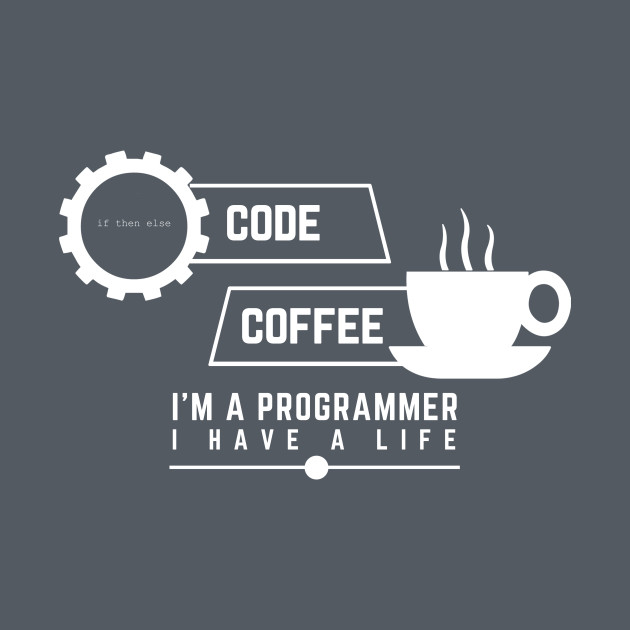# Circular rotation of an array using deque in C++

Given an array arr[] of integers and another integer D, the task is to perform D circular rotations on the array and print the modified array.

Examples:

Input: Arr[] = {1, 2, 3, 4, 5, 6}, D = 2
Output: 5 6 1 2 3 4

Input: Arr[] = {1, 2, 3}, D = 2
Output: 2 3 1

Approach:
Using Deque in C++, a rotation can be performed deleting the last element from the deque and inserting it at the beginning of the same deque.

Similarly, all the required rotations can be performed and then print the contents of the modified deque to get the required rotated array.

If the number of rotations is more than the size of the deque then just take the mod with N, So, D = D%N.
Where D is the number of rotations and N is the size of the deque.

Below is the implementation of the above approach:

 `// C++ implementation of the approach ` `#include ` `using` `namespace` `std; ` ` `  `// Function to circular rotate ` `// the array by d elements ` `void` `rotate(deque<``int``> deq, ` `            ``int` `d, ``int` `n) ` `{ ` `    ``// Push first d elements ` `    ``// from last to the beginning ` `    ``for` `(``int` `i = 0; i < d; i++) { ` `        ``int` `val = deq.back(); ` `        ``deq.pop_back(); ` `        ``deq.push_front(val); ` `    ``} ` ` `  `    ``// Print the rotated array ` `    ``for` `(``int` `i = 0; i < n; i++) { ` `        ``cout << deq[i] << ``" "``; ` `    ``} ` `    ``cout << endl; ` `} ` ` `  `// Driver code ` `int` `main() ` `{ ` `    ``deque<``int``> v = { 1, 2, 3, 4, 5, 6, 7 }; ` `    ``int` `n = v.size(); ` `    ``int` `d = 5; ` ` `  `    ``rotate(v, d % n, n); ` `} `

Output:

```3 4 5 6 7 1 2
```

My Personal Notes arrow_drop_upCheck out this Author's contributed articles.

If you like GeeksforGeeks and would like to contribute, you can also write an article using contribute.geeksforgeeks.org or mail your article to contribute@geeksforgeeks.org. See your article appearing on the GeeksforGeeks main page and help other Geeks.

Please Improve this article if you find anything incorrect by clicking on the "Improve Article" button below.

Article Tags :
Practice Tags :

Be the First to upvote.

Please write to us at contribute@geeksforgeeks.org to report any issue with the above content.